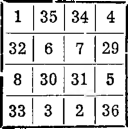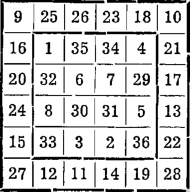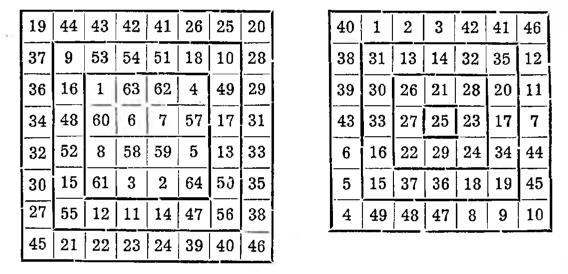# Squares with a border

Squares with a border. They are squares, which remain magical, although we will subtract one or more of the borders made of squares that run along the outer rows and columns. The method of construction given here can be applied to all squares and gives a great number of variations.

Take, for example, a magic square of the sixth order and set a target, that the square has one border, it means, so that the square of the fourth row in it remains magic after removing the border.

We will set up 36 first numbers as follows:
1 2 3 4 5 6 7 8 9 10 11 12 13 14 15 16 17 18
36 35 34 33 32 31 30 29 28 27 26 25 24 23 22 21 20 19

We are going to build a square of fourth order out of any eight numbers on line one and eight complementary numbers on line two, e.g:

1, 2, 3, 4, 5, 6, 7, 8 i 29, 30, 31, 32, 33, 34, 35, 36.We get a square with a magic sum 74. A square of the sixth row, which we were going to build, it has a magic sum, as we already know: 111. Hence the conclusion, that for each column, to each row and to each diagonal we should add two numbers, which in total give 37 = 111 — 74; but just this number 37 they give the numbers of the first and second lines listed above, when we sum them up in pairs. So we take the numbers that stand one after the other 9 i 28, 10 i 27 and place them in the corners of the square (6 X 6) so, that on the diagonals they complement each other to 37. We know now, that in the first row, four empty spaces should contain numbers, which in total will give
111 — (9 + 10) = 92, in the first column, the sum of the numbers inserted should be 111 — (9 + 27) = 75.From numbers, that are left to us, namely:

11 12 13 14 15 16 17 18

26 25 24 23 22 21 20 19

for the sum 92 we find as components, for example, numbers 26, 25, 23, 18. Let's put them in any order in the first row, and in the last row - their complement. Among the rest of the numbers, we further select four components that give the sum 75, so 16, 20, 24, 15 and put them in the first column, and their complement - in the last one. This gives a complete sixth row magic square with a border.
It is even easier to build such a square, when we take no to compose the central square 8 first and 8 the last numbers of the series, but the middle numbers:

11, 12, 18 i 19, …, 26.

To build a square of the eighth row, we do exactly the same: we are building a square 4 X 4 and surround it with a border; and having obtained the square 6X6, we frame it again; we then come to the square 8X8.

The same method can be used for odd squares. If we would like, for example,. build a square 7 X 7 with border, then we build a 3X3 square forward, we add a border to it, we get a square 5 X 5, and by adding a second border, we will obtain the forty-five-field square we are looking for.